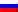Contenus

## Corrected exercises about graph theory and coloring

This page contains various corrected exercises about graph theory modelling and coloring problems. Please see graph theory page to learn about graph theory basics and various graph theory’s problem.

## Exercise 1

Considering a domino game using the numbers 0, 1, 2, 3, 4, as on each domino include two distinct digits, such as 1 and 3, the following problem is proposed: Is it possible to align all the dominoes so that when two pawns « touch » the numbers « in contact » are identical?

Show the problem as a complete graph K5. The problem is to find an Eulerian cycle in the graph.## Exercise 2

Consider the sequence 01110100 as being arranged in a circular pattern. Notice that every one of the eight possible binary triples: 000, 001, 011, . . . , 111 appear exactly once in the circular list. Can you construct a similar list of length 16 where all the four binary digit patterns appear exactly once each?

It is a graph with four vertices, each labeled with one of the possible pairs of binary digits. Imagine that each node is the last two digits in the pattern so far. The arrows leading away from a vertex are labeled either 0 or 1: the two possible values for the next digit that can be added to the pattern, and the ends of the arrows indicate the new final two digits. For example, node 00 go to 01 with an arc named 1, and go to 00 with an arc named 0.

To achieve every possible three-digit combination, we need to traverse the graph with an Eulerian cycle. Because of the result of the previous problem, there are always two arrows out and two arrows into each vertex, and because of the result of the previous problem, there must be an Eulerian circuit.

The situation is the same for any number of digits except that the graph will become more and more complex. For the 4-digit version, there will be 8 vertices and 16 edges, and so on. But in every case, there will be two edges entering and two leaving each vertex, so an Eulerian circuit is possible.## Exercise 3

A server can route a maximum of  packages at the same time. Seven substations are linked to a server, a substation cannot send packages if some substations already use the link. The next table presents each substation of the ability to send a package in function of the other ones. For example, a package from A cannot be sent if there is already a package from D but can be sent when B sent a package.

 Substation A B C D E F G Is not allowed with D,E D,E,F,G E,G A,B,E A,B,C,D,F,G B,E,G B,C,E,F

Represent the links in a graph. How many packages the server has to manage at the same time (maximum value)?The graph is 4-chromatic.

## Exercise 4

A school must pass written tests to four students: Pierre, Jean, Guillermo and Ibrahim. Seven disciplines are involved: mathematics, physics, biology, French, English, Spanish and history.
Pierre must pass mathematics, physics and English, Jean mathematics, biology and French, Guillermo mathematics, English and Spanish and Ibrahim physics, French and history.
What is the minimum amount of time slots to be expected for no student has to pass two tests simultaneously? What is the chromatic number of a complete graph? How to bound chromatic numbers in a graph?

A vertex represents a discipline, an edge links two disciplines if they cannot take place at the same time. The problem can be solved by searching a minimum number of colors. The largest complete sub graph is K3, so, there are at least 3 colors. The maximum degree is five, so there are at most 5 colors. By experimentation, the graph is 3-chromatic.FRFRENRUES